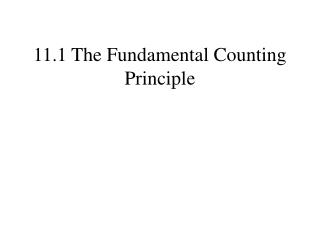DownloadDownload Presentation11.1 The Fundamental Counting Principle

# 11.1 The Fundamental Counting Principle

Télécharger la présentation## 11.1 The Fundamental Counting Principle

- - - - - - - - - - - - - - - - - - - - - - - - - - - E N D - - - - - - - - - - - - - - - - - - - - - - - - - - -
##### Presentation Transcript

1. 11.1 The Fundamental Counting Principle

2. What to wear? How many different ways can a young lady dress if she owns two pairs of pants and three blouses? There are three possibilities with the blue pants. There are also three possibilities with the brown? pants. There are a total of six possibilities (3 𝗑 2.)

3. What to eat? How many different ways can a meal be served if the entrée could be chilesrellenos, enchiladas, tacos, or tamales and the dessert could be cake, pie, or ice cream? There are three possibilities with the chilesrellenos. There are also three possibilities with the enchiladas, three with the tacos, and three with the tamales. There are a total of twelve possibilities (4 𝗑 3.)

4. The Fundamental Counting Principle How many different ways can a the letters A, and B be placed with the Greek letters Σ, Δ, and Θ and with the numbers 1, 2, 3, 4 and 5? The number of ways in which a series of successive things can occur is found by multiplying the number of ways in which each thing can occur. 2 𝗑 3 𝗑 5 = 30 A B ΣΔΘ ΣΔΘ 1 2 3 4 5 1 2 3 4 5 1 2 3 4 5 1 2 3 4 5 1 2 3 4 5 1 2 3 4 5

5. The Fundamental Counting Principle The number of ways in which a series of successive things can occur is found by multiplying the number of ways in which each thing can occur. A pizza can be ordered with thin, thick , or regular crust. You have a choice of ground beef, sausage, pepperoni, Canadian bacon, pineapple, or mushrooms. The pizza is available in large or medium sizes. How many different one topping pizzas can be ordered? 3 choices of crust 𝗑 6 choices of topping 𝗑 2 choices of size. 36 different ways of ordering.

6. The Fundamental Counting Principle A multiple choice test has 20 questions with 5 possible answers to each question. How many different ways can the answers be given. Two Correctly or incorrectly. There is only one correct way of answering all the questions. There are 520 − 1 or 95,367,431,640,624 ways of answering at least one of the questions incorrectly.

7. The Fundamental Counting Principle A multiple choice test has 15 questions with 4 possible answers to each question and 5 true/false questions. How many different ways can the answers be given. There are 415 ways of answering the four choice questions and 25 ways of answering the true/false questions. There are 415 𝗑 25 ways of answering the questions on the test. 34,359,738,368 different ways.

8. The Fundamental Counting Principle A multiple choice test has 15 questions with 4 possible answers to each question and 5 true/false questions. How many different ways can the answers be given. Last time we ignored the possibility of leaving a question blank. There are 515 ways of answering or not answering the four choice questions and 35 ways of answering or not answering the true/false questions. There are 515 𝗑 35 ways of answering or not answering the questions on the test. 7,415,771,484,375 different ways.

9. The Fundamental Counting Principle License plates are to be made with 2 letters followed by 4 numbers. How many different license plates are possible? 26 choices 10 choices 10 choices 26 choices 10 choices 10 choices There are 262 ways of using the letters and 104 ways of using the numbers. There are 262 𝗑 104 different license plates. 6,760,000 different license plates.